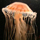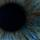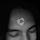## General Question# How to work out trig formulas in your head?

Asked by dotlin (419) July 22nd, 2010

My friend just looks at a trig formula and goes it’s close to X and will say what the answer is or close to the answer.

I didn’t ask him at the time but I want to know how to do this.

Observing members: 0Composing members: 0Memorize and be able to recreate this.

ipso (4466)“Great Answer” (0) Flag as…When x is close to zero, sin(x) and tan(x) are close to the radian measure of x

It is not hard to visualize this to get an intuitive feel for it. Imagine the unit circle centered at the origin of a grid. Draw a radius from the center to the circumference that makes a small angle. Drop a line from the point of the circle at the end of the radius to the x axis. The height of this line is the sine. Because the angle is small, the length of the line is close to the length of the circular arc from the x axis to the point, and this length is the radian measure of the angle.

LostInParadise (25444)“Great Answer” (1) Flag as…To add to what I said, you can use the idea to approximate cos and cot for x near pi/2 by use of the formula cos(x)=sin(pi/2x)

LostInParadise (25444)“Great Answer” (1) Flag as…The video ipso posted looks pretty neat. Here’s a similar idea: check out an image of a unit circle. Remember that the x coordinates around the edge are cosine values and the y coordinates are sine values of the angle inside the circle. When you’re given an angle, try to recreate the image of that unit circle in your head. There’s a lot of symmetries in it (e.g., just switching signs when the angle is larger than 90, 180, or 270 and sine and cosine are switched between 30 and 60) so it should be pretty quick to memorize after studying for a bit.

lapilofu (4325)“Great Answer” (1) Flag as…I still don’t get it so lets say I I’m working out sin(θ)= o/h reply and fill in θ, opposite and hypotenuse and explain what I would have to do, do the same with cos and tan.

dotlin (419)“Great Answer” (0) Flag as…Like I said, if sin(x) is small then x=sin(x), for x in radians. Additionally, it is easy to remember that sin(30 degrees) = ½. Just take an equilateral triangle and divide it into two triangles.

LostInParadise (25444)“Great Answer” (0) Flag as…or